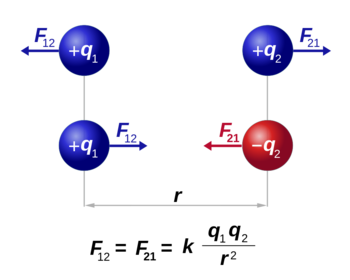# Coulomb's law

(diff) ← Older revision | Latest revision (diff) | Newer revision → (diff)Like charges have a force that pushes them further apart, unlike particles are atractive.

Coulomb's law states that "like charges repel, and unlike charges attract," but the more complete form determines the strength of the Coulomb force; Coulomb's law shows how strong the push or pull (the force) is between two points of charge, like a proton and electron in an atom. Coulomb's law is the simplest case of the electromagnetic force, one of the four fundamental forces. Most everyday forces like friction, the normal force, and air drag are residual forms of the Coulomb force (all of the charges add up to a complicated situation that leaves the friction between two surfaces, for example). Mathematically, Coulomb's law is very similar to Newton's law of gravity:

• is the force between the charges
• is Coulomb's constant and is 8.99 x 109 N m2/C2; this is related to the permittivity of free space
• and are the two charges (in coulombs)
• is the distance between the charges (in meters)

The law states that the electric force of one charge on another depends on the magnitude of the charges, and the square of the distance r between them.

The electric force pushing two protons in a nucleus apart is enormous compared to the gravitational force, about 1041 times larger (that's 100 000 000 000 000 000 000 000 000 000 000 000 000 000x larger). Coulomb's law inside the nucleus of atoms is what led scientists to postulate the strong force to hold the nuclei together, which is even stronger than the Coulomb force at short distances. This very short range of the strong nuclear force is what makes it so difficult to derive energy from nuclear fusion on Earth. The pushing apart of protons creates what's known as the Coulomb barrier.